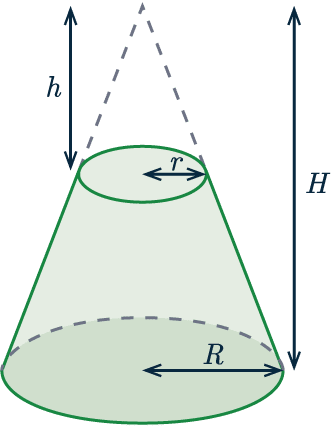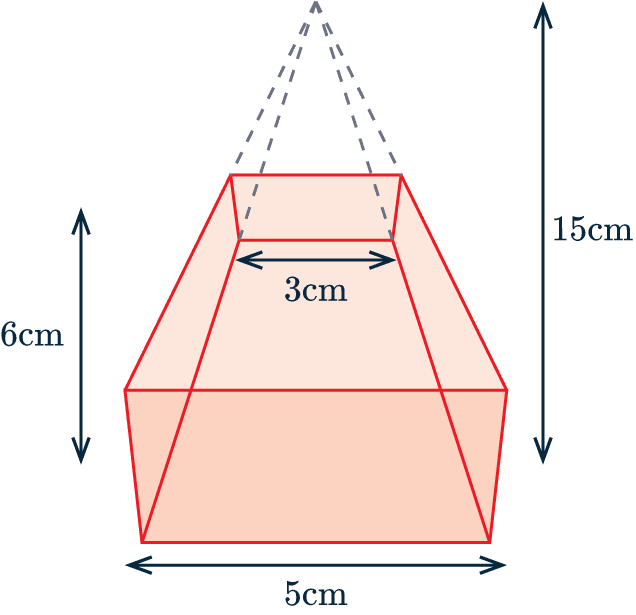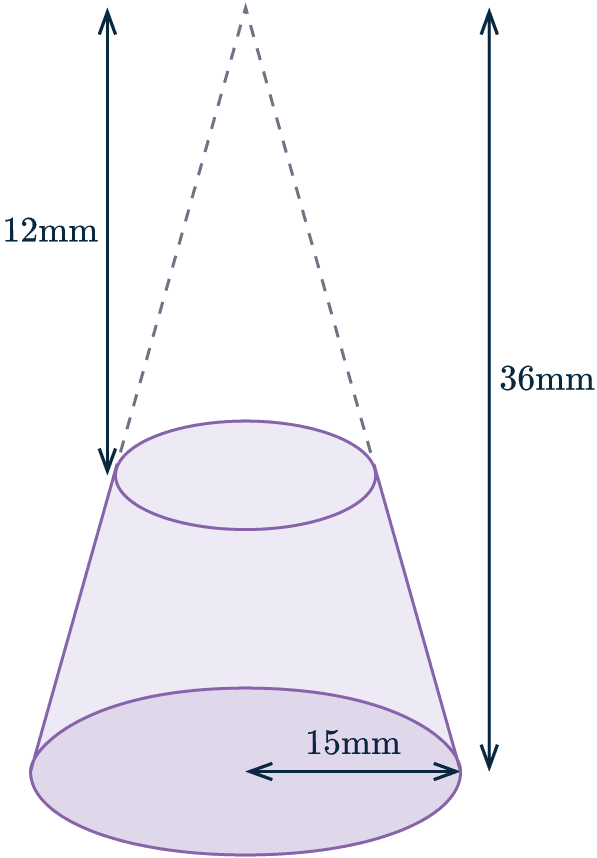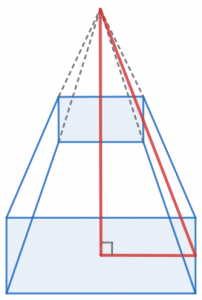# Frustums

GCSELevel 6-7AQAEdexcelOCRWJEC

## Frustums

The frustum of a cone or a pyramid is the 3D shape that is left-over after you cut the top off (see the pictures below for examples). The slice the you make when cutting the top off will always be parallel to the base of the shape.

Make sure you are happy with the following topics before continuing:

Level 6-7GCSEAQAEdexcelOCRWJEC

## Volume of Frustums

The volume of a frustum is calculated by first calculating the volume of the original cone or pyramid, then subtracting the volume of the portion removed.

This can be seen in the equation as follows:

$\textcolor{limegreen}{\text{Volume of a frustum}} = \textcolor{blue}{\text{Volume of original cone}} - \textcolor{red}{\text{Volume of cone removed}}$

Take a look at the image shown:

$\textcolor{limegreen}{V} = (\textcolor{blue}{\dfrac{1}{3}\pi\times R^2 \times H}) - (\textcolor{red}{\dfrac{1}{3}\pi \times r^2 \times h})$

The cone removed will always be mathematically similar to the original cone.Level 6-7GCSEAQAEdexcelOCRWJEC

## Example 1: Pyramid Frustum

Below is a frustum of a square-based pyramid.

The height of the original square-based pyramid is $15$cm and the height of the frustum is $6$cm.

Calculate the volume of the frustum.

[3 marks]The formula for the volume of a pyramid is

$\frac{1}{3}\times\textcolor{red}{\text{ area of base }}\times\textcolor{blue}{\text{ height}}$

We can calculate the volume of the whole pyramid (without the top chopped off) to be

$\dfrac{1}{3} \times \textcolor{red}{5}^2 \times \textcolor{blue}{15} = 125\text{ cm}^3$

Next, the volume of the missing pyramid, the height will be $15 - 6 = 9$cm and base $3$cm.

So we can calculate:

$\dfrac{1}{3} \times 3^2 \times 9 = 27\text{ cm}^3$

So, for the volume of the frustum, we subtract the smaller from the larger:

$125-27=98\text{ cm}^3$

Level 6-7GCSEAQAEdexcelOCRWJEC

## Example 2: Cone Frustum

Below is a frustum of a cone.

The height of the cone was originally  $36$ mm, and the height of the missing portion of the cone is $12$ mm.

The radius of the cone is $15$ mm.

Work out the Volume of the frustum. Leave the answer in terms of $\pi$.

[3 marks]First we need to calculate the volume of the original cone, we do this as follows.

$\dfrac{1}{3} \pi \times 15^2 \times 36 = 2700 \pi\text{ mm}^3$

Next we need to calculate he volume of the missing cone, to do this we need to find the radius of the smaller cone.

We know the smaller cone and larger cone are mathematically similar. This means the ratio of the height will be the same for both cones, meaning we can calculate the following:

$\dfrac{12}{36} = \dfrac{r}{15}$

Now we solve for $r$

$\dfrac{12}{36} \times 15 = r$

$r = 5\text{ mm}$

Using the value of $r$ we can calculate the volume of the smaller cone:

$\dfrac{1}{3} \pi \times 5^2 \times 12= 100\pi \text{ mm}^3$

Finally we calculate the volume of the frustum:

$V = 2700\pi - 100\pi = 2600\pi \text{ mm}^3$

Level 6-7GCSEAQAEdexcelOCRWJEC

## Frustums Example Questions

The volume of a cone is $\dfrac{1}{3}\pi r^2 h$. To find the volume of the frustum we need the volume of the whole cone and the volume of the missing portion. To calculate the latter, we will need the radius of the top of the frustum by using similarity.

The whole cone and the missing portion are similar. To find the scale factor between the dimensions of these two shapes, we must divide the height of one by the height of the other. The height of the missing portion is $50-30=20\text{cm}$, so the scale factor is $50\div20 = 2.5$. Therefore, the radius of the top face of the frustum is $10\div 2.5 = 4\text{cm}$. So, we get:

$\text{Volume of original cone}=\dfrac{1}{3}\pi\times 10^2\times 50=\dfrac{5000}{3}\pi$

$\text{Volume of missing portion}=\dfrac{1}{3}\pi\times 4^2\times 20=\dfrac{320}{3}\pi$

Finally, we get:

$\text{Volume of frustum} = \dfrac{5000}{3}\pi - \dfrac{320}{3}\pi = 4,900 \text{cm}^3$Gold Standard Education

To work out the surface area of this frustum we need the area of both the top square and the bottom square as well as the 4 trapezia that form the sides of the frustum. Since the apex is directly above the centre of the base, all these trapezia will be identical.

Working out the area of the squares is easy. The formula for the area of a trapezium is

$\dfrac{1}{2}(a + b)h$

where $a$ and $b$ are the two parallel sides. In this case, we know the two parallel sides are lengths 4m and 8m, but we don’t know the perpendicular height of each trapezium face. To do this, we’re going to need to use 3D trigonometry.

By constructing a right-angled triangle between the apex, the centre of the base, and the centre of one of the base’s sides, we can find the height of one of the triangular sides of the whole pyramid.We know that the base of this triangle will be half the base of the whole pyramid, so 4m, and we know the height is 7.5m. So, using Pythagoras, we get:

$c^2 = 4^2 + 7.5^2 \text{ therefore } c = \sqrt{72.25} = 8.5\text{m}$

The whole pyramid and the missing portion are similar shapes. Given that the base of one has width 8m and the base of the other has 4m, the scale factor is clearly 2. Therefore, now we know that the perpendicular height of one of the triangular faces of the whole pyramid is 8.5m, we can calculate the perpendicular height of one of the trapezoidal faces of the frustum:

$8.5 \div 2 = 4.25\text{m}$

Therefore, we get:

$\text{Area of trapezium} = \dfrac{1}{2}(4 + 8)\times 4.25 = 25.5\text{ m}^2$

To get the whole area, we must add 4 of these areas to the areas of the top face and the bottom face. We get:

$\text{Total area } = (4 \times 25.5) + 4^2 + 8^2 = 182\text{ m}^2$Gold Standard Education

The whole cone and the missing portion are similar shapes. Given that the base of one has a radius of 7 cm and the base of the other has a radius of 3 cm, the scale factor is, $3 \div 7 = \dfrac{3}{7}$

Therefore the height of the smaller cone is:

$10 \times \dfrac{3}{7} =\dfrac{30}{7} \text{cm}$

Thus, subtracting the height of the smaller cone from that of the original we are left with:

$\text{Height of frustum}=10 -\dfrac{30}{7} \approx 5.71 \text{cm}$Gold Standard Education

The whole cone and the missing portion are similar shapes. Given that the base of one has a radius of 12 cm and the base of the other has a radius of 3 cm, the scale factor is, $12 \div 3 = 4$

Therefore the slanted height of the smaller cone is:

$15 \div 4 = 3.75 \text{cm}$

Subtracting the slanted height of the smaller cone from the complete cone we are left the the slanted height of the frustum:

$\text{slanted height of frustum}= 15-3.75 = 11.25\text{cm}$Gold Standard Education

First we must find the volume of the complete triangular pyramid using the formula,

$\text{volume}=\dfrac{1}{3}\times \text{base area}\times \text{height}$.

the area of a triangle can be calculated using $\dfrac{1}{2}\text{ab}\sin{(c)}$, which in the case of equilateral triangle where all three sides are the same and each interior angle is 60 degrees, this becomes,

\begin{aligned} \dfrac{1}{2}\text{ab}\sin{(c)} &= \dfrac{1}{2}\text{a}^2\sin{(60)} \\ &= \dfrac{1}{2}\times a^2 \dfrac{\sqrt{3}}{2} = \dfrac{\sqrt{3}}{4} a^2\end{aligned}

In this instance $a = 11 \text{cm}$, thus

$\text{base area}=\dfrac{\sqrt{3}}{4}\times 11^2$

The height of the original pyramid is $20 \text{cm}$ so,

$\text{volume of complete pyramid}=\dfrac{1}{3}\times\dfrac{\sqrt{3}}{4}\times 11^2 \times 20 =349.3\text{cm}^3$

The volume of the smaller pyramid that is removed from the top can be calculated in much the same way:

$\text{volume of top pyramid}=\dfrac{1}{3}\times \dfrac{\sqrt{3}}{4}\times 7^2 \times 14 = 99 \text{cm}^3$

Subtracting the volume of the smaller pyramid from the complete pyramid we are left the the volume of the frustum:

$\text{volume of frustum}= 349.3 - 99 = 350.3\text{cm}^3$Gold Standard Education

## Frustums Worksheet and Example Questions

### (NEW) Frustums Exam Style Questions - MME

Level 6-7GCSENewOfficial MMEGold Standard Education

£19.99 /month. Cancel anytime

Level 4-5GCSE

Level 6-7GCSEKS3